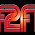CipherFeb 9, 2017

Super Bowl 51 - Bill Nye commercial and Trump - absolutely ridiculous coding!

On the day of Super Bowl 51 they released commercial for Persil featuring Bill Nye The Science Guy. We are gonna examine everything they gave us from this commercial
Before diving into deeper coding I will explain the simple part of why Bill Nye was chosen for the commercial, this is also connected to Trump in interesting way as I will explain below
His first name is William
William Nye = 5+9+3+3+9+1+4 + 5+7+5 = 51 (Reduced)
How convenient that he's showing up on Super Bowl 51

Bill Nye = 28+9+12+12 + 40+25+5 = 131 (Francis Bacon)
Super Bowl = 19+21+16+5+18 + 2+15+23+12 = 131 (Ordinal)
Championship = 3+8+1+13+16+9+15+14+19+8+9+16 = 131 (Ordinal)
Bill Nye of course has the same name gematria as the product he's advertising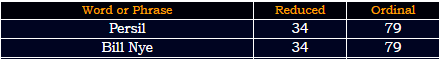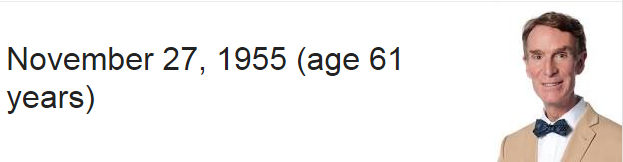November 27th is the date that leaves 34 days in the year
Bill Nye previously worked for Cornell University and Boeing
Boeing = 2+6+5+9+5+7 = 34 (Reduced)
Cornell = 3+6+9+5+5+3+3 = 34 (Reduced)
Bill Nye almost always appears with a "bowtie" = 7+3+4+7+9+4 = 34 (Reverse Reduced)
Note that Patriots won with 34 points
Falcons = 6+1+3+3+6+5+10 = 34 (S Exception)
Falcons = 6+1+3+3+6+5+1 = 25 (Reduced) - it was played on 2/5

Super Bowl Fifty one = 19+21+16+5+18 + 2+15+23+12 + 6+9+6+20+25 + 15+14+5 = 231 (Ordinal)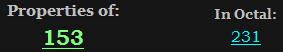The score was 34-28
Thirty four to twenty eight = 20+8+9+18+20+25 + 6+15+21+18 + 20+15 + 20+23+5+14+20+25 + 5+9+7+8+20 = 351 (Ordinal)

Bill Nye also has upcoming show which might be the reason they are promoting him at this time, the show is called "Bill Nye Saves The World" and it airs on 4/21/2017 (111th day in the year)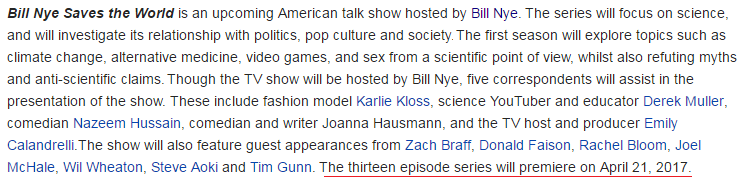Link to the trailer - Bill Nye Saves The World

Persil commercial aired on 2/5/2017 and it has corresponding gematria to the premiere date of this show
2/5/20172+5 + 2+0+1+7 = 17
4/21/2017 - 4+2+1 + 2+0+1+7 = 17
Super Bowl commercial was aired 71 days after his birthday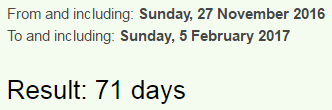From Super Bowl to the release of his show is also 75 days (2 months, 16 days - 6*6*6 = 216)61 is 75 in octal numbering system, he is currently 61 years old and his name gematria points to 61 as well
Thirty Four = 2+8+9+9+2+7 + 6+6+3+9 = 61 (Reduced)It's unbelievable how they announced the show on 31st of August (Thirty first of August = 93 R).....In Europe this date would be written 31/8 which reminds a lot of 318 and it comes 2 months and 27 days before his birthday (88 days), this 227 coding blows me away for many reasons connecting to Trump(88) as I will explain shortly22/7 = 3.14 - Pi
7/22 = 0.318 - 'Inverse Pi'
Twenty two divided by seven = 20+23+5+14+20+25+20+23+15+4+9+22+9+4+5+4+2+25+19+5+22+5+14 = 314 (Ordinal)
The most ridiculous part is when we measure to the release of the show and find reflection - it comes 7 months and 22 days before the release of his TV show...also it comes 234 days (234+432 = 666) and 33 weeks and 3 days later..The name of the show has the same gematria as Trump and it's no coincidence that Super Bowl 51 comes 88 days after he won the election vote on 11/9/2016 - (2 months, 27 days)Bill Nye Saves The World = 2+9+3+3 + 5+7+5 + 1+1+4+5+1 + 2+8+5 + 5+6+9+3+4 = 88 (Reduced)
Trump = 20+18+21+13+16 = 88 (Ordinal)
This could very well be one of the clues for Trump's assassination

It's interesting that Pi sums to 25 considering all the 227 coding around Super Bowl and Trump
Pi = 16+9 = 25 (Ordinal)
Trump = 2+9+3+4+7 = 25 (Reduced) - a lot like the day of Super Bowl on 2/5
Bill Nye The Science Guy = 2+9+3+3+5+7+5+2+8+5+1+3+9+5+5+3+5+7+3+7 = 97 (Reduced) - 25th prime number is 97
Bill Nye Saves The World = 2+9+12+12 + 14+25+5 + 19+1+22+5+19 + 20+8+5 + 23+15+18+12+4 = 250 (Ordinal)
Note that Super Bowl 51 also comes 7 months and 22 days after Trump's birthday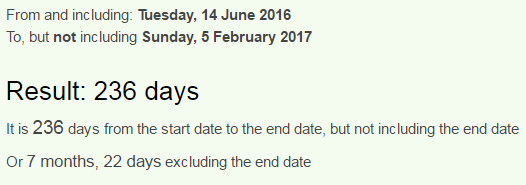So going back to the commercial, they ended it with very interesting phrases that seem to be coded out very well
It ends with this sceneLet's examine the writing here
Its not just clean = 9+2+1 + 5+6+2 + 1+3+1+2 + 3+3+5+1+5 = 49 (Reduced)
Its not just clean = 9+200+100 + 50+60+200 + 10+300+100+200 + 3+30+5+1+50 = 1318 (English)
When I look at these numbers I see Revelation 13:18, hopefully I will do a decent job explaining this part
Revelation = 9+5+4+5+3+1+2+9+6+5 = 49 (Reduced) - 49th prime number is 227
On the top they write "10 Dimensions" - Ten Dimensions = 61, Persil = 34, Thirty four = 61, God = 61 (Jewish)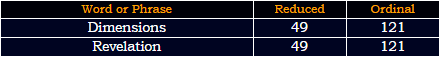The last part is very key to this riddle
Its proclean = 9+2+1 + 7+9+6+3+3+5+1+5 = 51 (Reduced)
Its proclean = 9+20+19 + 16+18+15+3+12+5+1+14 = 132 (Ordinal)
First we see 51 a lot like Super Bowl 51, but I think there is deeper meaning to this
This 51 might be pointing to 5/1 (May first) because it comes 2 months and 27 days from Super BowlFirst of May has exactly the same gematria as "It's proclean", this is historically a very special date and this year there might be a major false flag event taking place in the USA
First of May = 6+9+9+1+2 + 6+6 + 4+1+7 = 51 (Reduced)
First of May = 6+9+18+19+20 + 15+6 + 13+1+25 = 132 (Ordinal)

Revelation = 18+5+22+5+12+1+20+9+15+14 = 121 (Ordinal)May First = 78+6+150 + 36+54+108+114+120 = 666 (Sumerian)
May First = 4+1+7 + 6+9+9+10+2 = 48 (S Exception)
Donald Trump = 4+6+5+1+3+4 + 2+9+3+4+7 = 48 (Reduced)
The verse they are pointing to is the verse that talks about 666 - "number of the beast/man", examine this picture carefully and note how Bill Nye The Science Guy and Super Bowl 51 has extremely interesting gematria connecting to a lot of stuff on the picture
Super Bowl Fifty One = 90+200+60+5+80 + 2+50+900+20 + 6+9+6+100+400 + 50+40+5 = 2023 (Jewish)
Bill Nye The Science Guy = 2+9+12+12 + 14+25+5 + 20+8+5 + 19+3+9+5+14+3+5 + 7+21+25 = 223 (Ordinal)It's very interesting how 13*18 gives us another 666

Now here are other reasons I think something is coming up on 5/1/2017:
1. Trump's tombstone appeared in Central Park on March 27th, 2016, "artist" that made it was 33 years old and his name is "Brian Whiteley" - Brian = 44 (Ordinal), Whiteley = 44 (Reduction)
Twenty Seventh of March = 2+5+5+5+2+7 + 1+5+4+5+5+2+8 + 6+6 + 4+1+9+3+8 = 93 (Reduced)
Central Park = 3+5+14+20+18+1+12 + 16+1+18+11 = 119 (Ordinal)Tombstone appeared 227 days before Trump won the election vote on 11/9 (314th day in 2016 because it's a leap year) - 227? 314??
Donald = 4+50+40+1+20+4 = 119 (Jewish)
Mr Trump = 13+18 + 20+18+21+13+16 = 119 (Ordinal)
The reason there's insane amount of 227 and 119 coding surrounding Trump is his name itself and the connection between these 2 numbers through Saturn

2. In the TV show called "Lucifer" there is an episode called "Trip to stabby town" where Lucifer says this:

Lucifer: Yes, at least for now. So... we can talk about... Caligula, Stalin, Trump...I mean...I know he's not dead, but he's definitely going.

It came out on 11/14 leaving 47 days in the year (President = 47), but it doesn't end there with that crazy TV show (I made a post about this episode way back in November when it aired - Trump 5/1/2017)
Trump = 100+80+200+30+60 = 470 (Jewish)
Lucifer = 12+21+3+9+6+5+18 = 74 (Ordinal)
The episode Trip to Stabby town was released 153 days after his birthday (special number connecting to 318 and catch of 153 fish - 318 153 postAlso it is 21 weeks and 6 days a lot like 6*6*6 = 216The series went on a winter break on 11/28/2016 and aired the next episode on 1/16/2017 then after 2 episodes it went on a huge break until May First... the craziest part is the last episode that aired is called "A Good Day to Die"... how weird is that? ... Trip to stabby town? Gives a prediction and they go on a break after 'a good day to die'..
Also note that "A good way to die" came out on a date with 93 numerology just like 27th of March when Trump's tombstone appeared
Thirtieth of January = 2+8+9+9+2+9+5+2+8 + 6+6 + 1+1+5+3+1+9+7 = 93 (Reduced)
May = 13+1+25 = 39 (Ordinal)
May First = 4+1+7 + 6+9+9+1+2 = 39 (Reduced)

The episode is coming back with 14th episode on 5/1/2017
Fourteenth episode = 6+6+3+9+2+5+5+5+2+8 + 5+7+1+9+6+4+5 = 88 (Reduced)
Trump = 20+18+21+13+16 = 88 (Ordinal)
Winter break of the series lasted for 49 days like "Revelation" and 1 month, 19 days like the number of Saturn (119)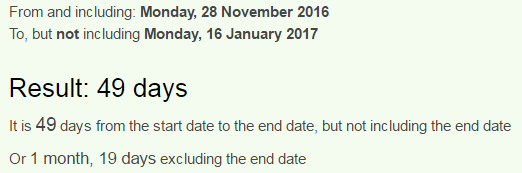I have a post decoding Bill Nye and his bullshit show from a few months back - The Actor Guy

Other posts with Trump assassination codes:
Artist - Bloody Mural Protest
My Way CNN coding

1.1.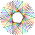2.